Saturday, July 11, 2020
Home > CBSE Class 12 > NCERT Solutions Class 12 Maths 3D Geometry Exercise 11.2

# NCERT Solutions Class 12 Maths 3D Geometry Exercise 11.2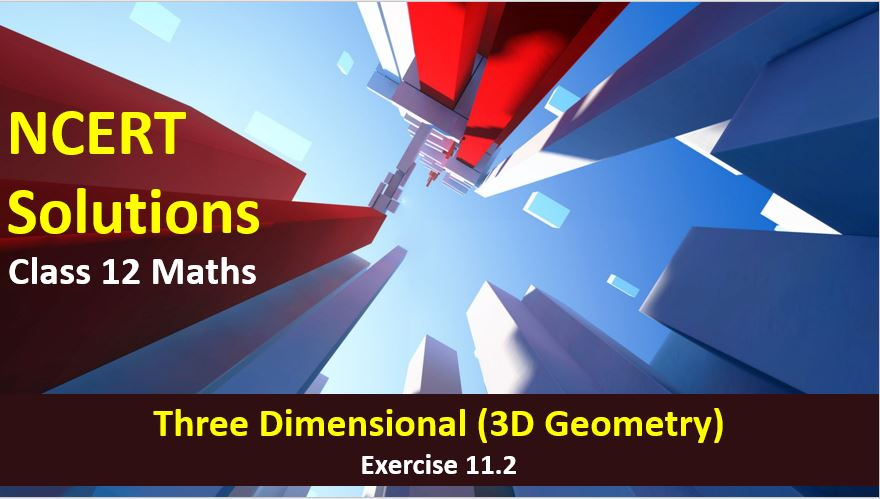# NCERT Solutions for Class 12 Maths 3D Geometry

Hi Students, Welcome to Amans Maths Blogs (AMB). In this post, you will get the NCERT Solutions for Class 12 Maths 3D Geometry Exercise 11.2.

As we know that all the schools affiliated from CBSE follow the NCERT books for all subjects. You can check the CBSE NCERT Syllabus. Thus, NCERT Solutions helps the students to solve the exercise questions as given in NCERT Books.

NCERT Solutions for class 12 is highly recommended by the experienced teacher for students who are going to appear in CBSE Class 12 and JEE Mains and Advanced and NEET level exams. Here You will get NCERT Solutions for Class 12 Maths Three Dimensional Geometry Exercise 11.2 of all questions given in NCERT textbooks of class 12 in details with step by step process.

The PDF books of NCERT Solutions for Class 12 are the first step towards the learning and understanding the each sections of Maths, Physics, Chemistry, Biology as it all help in engineering medical entrance exams. To solve it, you just need to click on download links of NCERT solutions for class 12.

CBSE Class 12th is an important school class in your life as you take some serious decision about your career. And out of all subjects, Maths is an important and core subjects. So CBSE NCERT Solutions for Class 12th Maths is major role in your exam preparation as it has detailed chapter wise solutions for all exercise. This NCERT Solutions can be downloaded in PDF file. The downloading link is given at last.

NCERT Solutions for Class 12 Maths are not only the solutions of Maths exercise but it builds your foundation of other important subjects. Getting knowledge of depth concept of CBSE Class 12th Maths like Algebra, Calculus, Trigonometry, Coordinate Geometry help you to understand the concept of Physics and Physical Chemistry.

## NCERT Solutions for Class 12 Maths 3D Geometry

Note: In this solution, the vector is represented by BOLD font. For example: a, b, OP, AB, i, j, k represent the vectors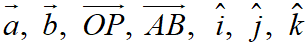NCERT Solutions for Class 12 Maths 3D Geometry Exercise 11.2 Ques No 1.

Show that the three lines with direction cosines (12/13, −3/13, −4/13); (4/13, 12/13, 3/13); (3/13, −4/13, 12/13); are mutually perpendicular.

NCERT Solutions:

Let the line L1 has direction cosines as l1 = 12/13, m1 = −3/13, n1 = −4/13.

Let the line L2 has direction cosines as l2 = 4/13, m2 = 12/13, n2 = 3/13.

Let the line L3 has direction cosines as l3 = 3/13, m3 = −4/13, n3 = 12/13.

Let the angle between the lines L1 and L2 is θ1. Then,

cos θ1 = |l1l2 + m1m2 + n1n2|

= |(12/13 x 4/13) + (−3/13 x 12/13) + (−4/13 x 3/13)|

= |48/169 − 36/169 −12/169|

= |(48 − 36 − 12)/169| = 0 ⇒ θ1 = 90

Thus, the angle between the lines L1 and L2 is 90o.

Let the angle between the lines L2 and L3 is θ2. Then,

cos θ2 = |l2l3 + m2m3 + n2n3

= |(4/13 x 3/13) + (12/13 x −4/13) + (3/13 x 12/13)|

= |12/169 − 48/169 + 36/169| = |(12 − 48 + 36)/169| = 0

⇒ θ2 = 90

Thus, the angle between the lines L2 and L3 is 90o.

Let the angle between the lines L1 and L3 is θ3. Then,

cos θ3 = |l1l3 + m1m3 + n1n3

= |(12/13 x 3/13) + (−3/13 x −4/13) + (−4/13 x 12/13)|

= |36/169 + 12/169 − 48/169| = |(36 + 12 − 48)/169| = 0

⇒ θ3 = 90

Thus, the angle between the lines L1 and L3 is 90o.

Therefore the given lines are mutually perpendicular.

NCERT Solutions for Class 12 Maths 3D Geometry Exercise 11.2 Ques No 2.

Show that the line through the points (1, – 1, 2), (3, 4, – 2) is perpendicular to the line through the points (0, 3, 2) and (3, 5, 6).

NCERT Solutions:

Direction ratios of the line joining the points A(1, – 1, 2) and B(3, 4, – 2) are

a1 = (3 – 1) = 2, b1 = (4 + 1) = 5, c1 = (–2 – 2) = –4

Direction ratios of the line joining the points C(0, 3, 2) and D(3, 5, 6) are

a2 = (3 – 0) = 3, b2 = (5 – 3) = 2, c2 = (6 – 2) = 4.

Now, two lines with direction ratios a1, b1, c1 and a2, b2, c2 are perpendicular if

a1a2 + b1b2 + c1c2 = 0

Since a1a2 + b1b2 + c1c2 = (2 x 3) + (5 x 2) + (–4 x 4) = 6 + 10 – 16 = 0, then the given lines AB and CD are perpendicular.

NCERT Solutions for Class 12 Maths 3D Geometry Exercise 11.2 Ques No 3.

Show that the line through the points (4, 7, 8), (2, 3, 4) is parallel to the line through the points (– 1, – 2, 1), (1, 2, 5).

NCERT Solutions:

Direction ratios of the line joining the points A(4, 7, 8) and B(2, 3, 4) are

a1 = (2 – 4) = –2, b1 = (3 – 7) = –4, c1 = (4 – 8) = –4

Direction ratios of the line joining the points C(– 1, – 2, 1) and D(1, 2, 5) are

a2 = (1 + 1) = 2, b2 = (2 + 2) = 4, c2 = (5 – 1) = 4.

Now, two lines with direction ratios a1, b1, c1 and a2, b2, c2 are parallel if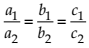Since, then the given lines AB and CD are parallel.

NCERT Solutions for Class 12 Maths 3D Geometry Exercise 11.2 Ques No 4.

Find the equation of the line which passes through the point (1, 2, 3) and is parallel to the vector 3i + 2j −2k.

NCERT Solutions:

Given that the line which passes through the point A(1, 2, 3). Then, a = i + 2j + 3k. And, the this line is parallel to the vector b = 3i + 2j −2k.

We know that the vector equation of the line passing through a and parallel to b is r = a + λb. where λ is any real number.

Thus, the required vector equation of the given line is r = (i + 2j + 3k) + λ(3i + 2j −2k).

NCERT Solutions for Class 12 Maths 3D Geometry Exercise 11.2 Ques No 5.

Find the equation of the line in vector and in Cartesian form that passes through the point with position vector 2ij + 4k and is in the direction i + 2jk.

NCERT Solutions:

Given that the line which passes through the point with position vector a = 2ij + 4k and is in the direction b = i + 2jk

We know that the vector equation of the line passing through a and parallel to b is r = a + λb. where λ is any real number.

Thus, the vector equation of the given line is r = (2ij + 4k) + λ(i + 2j − k).

Since r is the position vector of any point P(x, y, z), then r = xi + yj + zk.

Thus,

xi + yj + zk = (2ij + 4k) + λ(i + 2j − k)

xi + yj + zk = (2 + λ)i + (−1 + 2λ)j + (4 − λ)k

x = (2 + λ), y = (−1 + 2λ), z = (4 − λ)

Eliminating λ, we get the Cartesian equation of line asNCERT Solutions for Class 12 Maths 3D Geometry Exercise 11.2 Ques No 6.

Find the Cartesian equation of the line which passes through the point (– 2, 4, – 5) and parallel to the line given by.

NCERT Solutions:

Given that the line passes through the point P(– 2, 4, – 5) and parallel to the line given by.

Thus, the line passing through P(x1, y1, z1) where x1 = – 2, y1 = 4, z1 = – 5 and the direction ratios

a1 = 3, b1 = 5, c1 = 6 is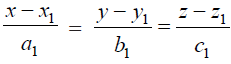NCERT Solutions for Class 12 Maths 3D Geometry Exercise 11.2 Ques No 7.

The cartesian equation of a line is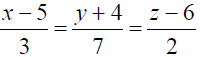. Write its vector form.

NCERT Solutions:

Comparing the given equationwith the standard form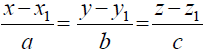We observe that x1 = 5, y1 = –4, z1 = 6; a = 3, b = 7, c = 2.

Thus, the required line passes through the point P(5, –4, 6) a = 5i – 4j + 6k and is parallel to the vector b = 3i + 7j +2k.

Let r be the position vector of any point on the line, then the vector equation of the line is given by

r = a + λb r = (5i – 4j + 6k) + λ(3i + 7j +2k).

NCERT Solutions for Class 12 Maths 3D Geometry Exercise 11.2 Ques No 8.

Find the vector and the Cartesian equations of the lines that passes through the origin and (5, – 2, 3).

NCERT Solutions:

Given that the line passes through the origin P(0, 0, 0) a = 0i + 0j + 0k and Q(5, – 2, 3) b = 5i – 2j + 3k.

Let r be the position vector of any point on the line, then the vector equation of the line is given by

r = a + λ(b – a)

⇒ r = (0i + 0j + 0k) + λ(5i – 2j + 3k – 0i – 0j – 0k)

⇒ r = 0 + λ(5i – 2j + 3k).

Now, put r = xi + yj + zk.

xi + yj + zk = λ(5i – 2j + 3k)

xi + yj + zk = (5λ)i + (−2λ)j + (3λ)k

x = (5λ), y = (−2λ), z = (3λ)

Eliminating λ, we get the Cartesian equation of line asNCERT Solutions for Class 12 Maths 3D Geometry Exercise 11.2 Ques No 9.

Find the vector and the Cartesian equations of the line that passes through the points (3, – 2, – 5), (3, – 2, 6).

NCERT Solutions:

Given that the line passes through the origin A(3, – 2, – 5) a = 3i – 2j – 5k and B(3, – 2, 6) b = 3i – 2j + 6k.

Let r be the position vector of any point on the line, then the vector equation of the line is given by

r = a + λ(b – a)

⇒ r = (3i – 2j – 5k) + λ(3i – 2j + 6k – 3i + 2j + 5k)

⇒ r = (3i – 2j – 5k) + λ(0i + 0j + 11k)

⇒ r = (3i – 2j – 5k) + λ(11k)

Now, put r = xi + yj + zk.

xi + yj + zk = (3i – 2j – 5k) + λ(11k)

xi + yj + zk = (3 + 0λ)i + (−2 + 0λ)j + (– 5 + 11λ)k

x = (3 + 0λ), y = (−2 + 0λ), z = (– 5 + 11λ)

Eliminating λ, we get the Cartesian equation of line as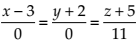NCERT Solutions for Class 12 Maths 3D Geometry Exercise 11.2 Ques No 10 (i).

Find the angle between the following pairs of lines (i) r = 2i − 5j + k+ λ(3i + 2j + 6k) and r = 7i − 6k + μ(i + 2j + 2k)

NCERT Solutions:

Given that b1 = 3i + 2j + 6and b2 = i + 2j + 2k.

Let θ be the angle between the given lines. Then,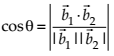NCERT Solutions for Class 12 Maths 3D Geometry Exercise 11.2 Ques No 10 (ii)

Find the angle between the following pairs of lines (i) r = 3i + j − 2k+ λ(ij − 2k) and r = 2ij − 56k + μ(3i − 5j − 4k).

NCERT Solutions:

Given that b1 = ij − 2k and b2 = 3i − 5j − 4k.

Let θ be the angle between the given lines. Then,NCERT Solutions for Class 12 Maths 3D Geometry Exercise 11.2 Ques No 11(i)

Find the angle between the following pair of lines

(i)NCERT Solutions:

Direction ratio of the given lines are a1 = 2, b1 = 5, c1 = -3 and a2 = -1, b2 = 8, c2 = 4.

Let θ be the angle between the given lines. Then,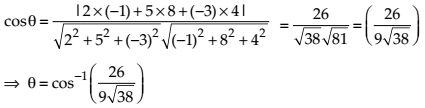NCERT Solutions for Class 12 Maths 3D Geometry Exercise 11.2 Ques No 12

Find the values of p so that the lines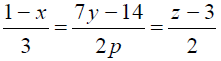and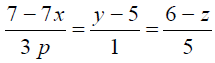at right angles.

NCERT Solutions:

Given equation can be written as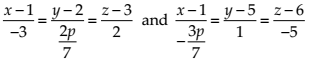Then, the direction ratios of the given lines are a1 = -3, b1 = 2p/7, c1 = 2 and a2 = -3p/7, b2 = 1, c2 = -5.

Two lines with direction ratios a1, b1, c1 and a2, b2, c2 are perpendicular, if a1a2 + b1b2 + c1c2 = 0.NCERT Solutions for Class 12 Maths 3D Geometry Exercise 11.2 Ques No 13

Show that the lines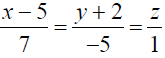and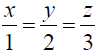are perpendicular to each other.

NCERT Solutions:

Given equation of the lines areand.

Then, the direction ratios of the given lines are a1 = 7, b1 = -5, c1 = 1 and a2 = 1, b2 = 2, c2 = 3.

Two lines with direction ratios a1, b1, c1 and a2, b2, c2 are perpendicular, if a1a2 + b1b2 + c1c2 = 0.

Now, a1a2 + b1b2 + c1c2 = (7)(1) + (-5)(2) + (1)(3) = 0. Thus, the given lines are perpendicular.

NCERT Solutions for Class 12 Maths 3D Geometry Exercise 11.2 Ques No 14

Find the shortest distance between the lines r = (i + 2j + k) + λ(ij + k) and r = 2ijk + μ(2i + j + 2k).

NCERT Solutions: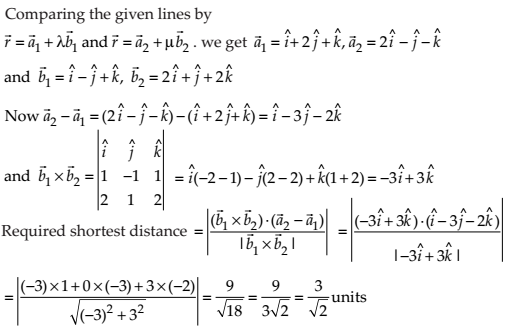NCERT Solutions for Class 12 Maths 3D Geometry Exercise 11.2 Ques No 15

Find the shortest distance between the lines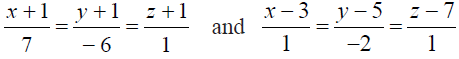NCERT Solutions:NCERT Solutions for Class 12 Maths 3D Geometry Exercise 11.2 Ques No 16

Find the shortest distance between the lines whose vector equations are r = (i + 2j + 3k) + λ(i − 3j + 2k) and r = 4i + 5j + 6k + μ(2i + 3j + k).

NCERT Solutions: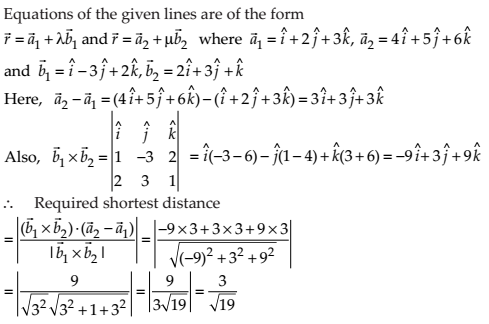NCERT Solutions for Class 12 Maths 3D Geometry Exercise 11.2 Ques No 17

Find the shortest distance between the lines whose vector equations are r = (1− t)i + (t − 2)j + (3 − 2t)k and r = (s +1)i + (2s − 1)j − (2s +1)k.

NCERT Solutions: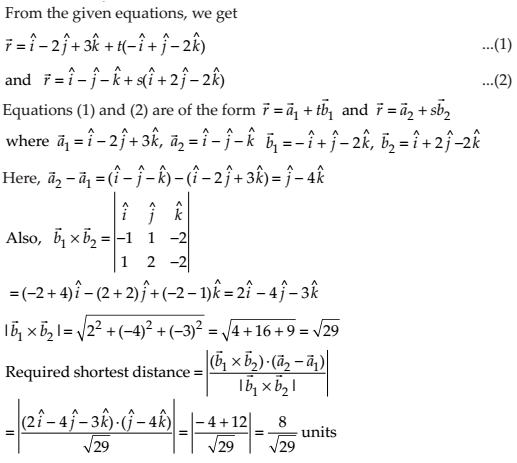Check Other Article Published on Amans Maths Blogs:

error: Content is protected !!